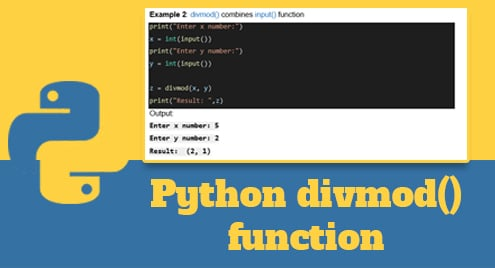Scripting

# Python divmod() function## Introduction

The divmod() function is used to divide and return the result as an integer and the remainder of the two entered numbers. This is a common math operation in python to help solve math problems. Maybe it will help a lot in the school’s math program.

And we will show you how to use the divmod() function in Python as you go through it below. Hope you understand.

## Example

```x = divmod(7, 2)

print(x)
```

Output:

`(3, 1)`

## Definition

The divmod() function returns 2 values: integer and remainder.

## The syntax

```divmod(x, y)
```

Parameter Values:

```x: dividend

y: divisor
```

## More examples

Example 1: Basic divmod()

```x = divmod(9, 4)

y = divmod(7, 2)

print("x = ", x)

print("y = ", y)
```

Output:

```x = (2, 1)

y = (3, 1)
```

Example 2:

```divmod() combines input() function

print("Enter x number:")

x = int(input())

print("Enter y number:")

y = int(input())

z = divmod(x, y)

print("Result: ",z)
```

Output:

```Enter x number: 5

Enter y number: 2

Result: (2, 1)
```

## Conclusion

Hope you understood the tutorial on how to use the divmod() function in Python.